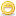1. ## Programming Question

Alright, I'm trying to learn Visual Basic, and I'm still a newbie so don't flame me for what's probably a stupid question.

I'm trying to make this program that tells you how long to cook a turkey. You're supposed to cook it 17 minutes for every pound. If it's 6 pounds, it would be 102 minutes, which is 1 hour and 42 minutes. When I run the program though, it gives me 2 hours and 18 minutes. I looked over the code and I cannot figure out what the problem is and it's kind of aggrevating me. If someone can look through and find what the problem is, I'd really appreciate it. Here's the code:

Dim intWeight As Integer
Dim intMinutes As Integer
Dim intHours As Integer
Dim intTime As Integer

Private Sub cmdCalculate_Click()
intWeight = txtWeight.Text
intTime = intWeight * 17
intHours = intTime / 60
intMinutes = intTime - intHours * 60

If intHours * 60 > intTime Then
intMinutes = intHours * 60 - intTime
End If

lblTime.Caption = "Cook your turkey for " & intHours & " hours and for " & intMinutes & " minutes."
End Sub

Private Sub txtWeight_KeyPress(KeyAscii As Integer)
cmdCalculate.Visible = True
End Sub

Thanks!2. ##rounding error

the problem is rounding error caused by the classification of one of the variables. You have "Dim intHours As Integer," which would be fine, if everything divided evenly by 60, because later in the code you have "intHours = intTime / 60."

Unfortunately, this results in 1.7, which is not an integer, so VB rounds it off as 2. From then on, the rest of the math and the print out is messed up because it will be using 2 instead of 1.7. Perhaps finding a way to cut off everything after the decimal would work? When/If I am able to create a fixed piece of code to resolve the problem, I'll post it here.

Hope this is what you were looking for3. ##got it

this small fix should fix the problem:

intHours = fix(intTime / 60)

*note: I have not tested this yet, but it should work

This should cut off the end and return only the integer part, which is what you need.

have fun4. Hey, thanks man. That actually makes sense. I fixed the code and it works now. I really appreciate your help.####Posting Permissions

• You may not post new threads
• You may not post replies
• You may not post attachments
• You may not edit your posts
•